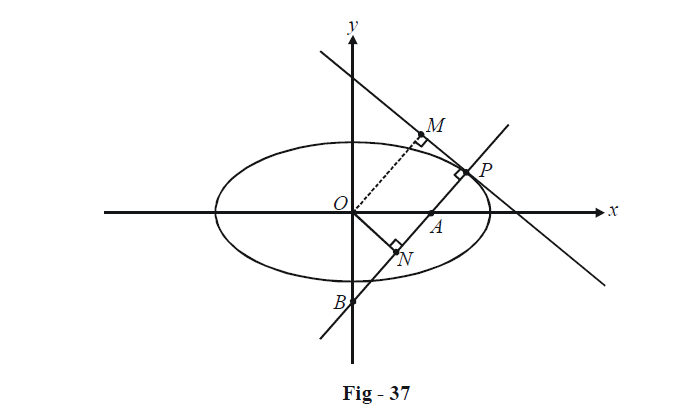# Examples On Normals To Ellipses Set-2

Go back to  'Ellipse'

Example – 34

The normal at any point P on the ellipse $$\frac{{{x^2}}}{{{a^2}}} + \frac{{{y^2}}}{{{b^2}}} = 1$$ meets the major and minor axes at A and B respectively. ON is the perpendicular upon this normal from the centre O of the ellipse. Show that

$PA \cdot PN = {b^2}andPB \cdot PN = {a^2}$

Solution:Assume the point P to be $$(a\cos \theta ,\,b\sin \theta ).$$ The normal at P has the equation

$(a\sec \theta )x - (b\,{\rm{cosec}}\,\theta )y = {a^2} - {b^2}\qquad\qquad...\left( 1 \right)$

The coordinates of A are therefore

$A \equiv \left( {\frac{{{a^2} - {b^2}}}{{a\sec \theta }},\,0} \right) \equiv (a{e^2}\cos \theta ,\,0)$

Similarly, B is

$B \equiv \left( {0,\,\,\frac{{{b^2} - {a^2}}}{{b\,{\rm{cosec }}\theta }}} \right) \equiv \left( {0,\,\,\frac{{ - {a^2}{e^2}}}{b}\sin \theta } \right)$

PA and PB can now be evaluated using the distance formula:

\begin{align}&\;\;PA = \sqrt {{{(a\cos \theta - a{e^2}\cos \theta )}^2} + {{(b\sin \theta )}^2}} \\&\qquad{\rm{ }} = \sqrt {\frac{{{b^4}}}{{{a^2}}}{{\cos }^2}\theta + {b^2}{{\sin }^2}\theta } \\&\qquad{\rm{ }} = \frac{b}{a}\sqrt {{a^2}{{\sin }^2}\theta + {b^2}{{\cos }^2}\theta } \qquad\qquad...\left( 2 \right)\\&\;\;PB = \sqrt {{{(a\cos \theta )}^2} + {{\left( {b\sin \theta + \frac{{{a^2}{e^2}}}{b}\sin \theta } \right)}^2}} \\&\qquad{\rm{ }} = \frac{a}{b}\sqrt {{a^2}{{\sin }^2}\theta + {b^2}{{\cos }^2}\theta }\qquad\qquad...\left( 3 \right)\end{align}

PN can be evaluated either using the perpendicular distance of O from the normal at $$P(P{N^2} = O{P^2} - O{N^2})$$ or simply as the perpendicular distance of O from the tangent at P.

The tangent at P has the equation

$bx\cos \theta + ay\sin \theta - ab = 0$

Thus,

$PN = \frac{{\left| {ab} \right|}}{{\sqrt {{b^2}{{\cos }^2}\theta + {a^2}{{\sin }^2}\theta } }}\qquad\qquad...\left( 4 \right)$

From (2), (3) and (4), we have

$PA \cdot PN = {b^2}\;and\;PB \cdot PN = {a^2}$

Example - 35

Prove that from any given point$$P(h,\,k),$$ four normals (real or imaginary) can be drawn to the ellipse $$\frac{{{x^2}}}{{{a^2}}} + \frac{{{y^2}}}{{{b^2}}} = 1$$ and the sum of the eccentric angles of the feet of these four normals is an odd integral multiple of $$\pi$$

Solution: Any normal to the ellipse is of the form

$(a\sec \theta )x - (b\,{\rm{cosec }}\theta )y = {a^2} - {b^2}$

If this passes through $$P(h,\,\,k),$$ we have

$ah\sec \theta - bk\,{\rm{cosec }}\theta = {a^2} - {b^2}\qquad...\left( 1 \right)$

We need to show that this equation will in general yield four values of $$\theta$$ For this purpose, we use the substitution

$\cos \theta \to \frac{{1 - {t^2}}}{{1 + {t^2}}},\,\,\,\sin \theta = \frac{{2t}}{{1 + {t^2}}}$

where $$t = \tan \frac{\theta }{2}.$$ Thus, (1) transforms to,

\begin{align}&ah\left( {\frac{{1 + {t^2}}}{{1 - {t^2}}}} \right) - bk\left( {\frac{{1 + {t^2}}}{{2t}}} \right) = {a^2} - {b^2} = {a^2}{e^2}(\because \,\,{b^2} = {a^2}(1 - {e^2})) \hfill \\&\Rightarrow \quad bk{t^4} + 2(ah + {a^2}{e^2}){t^3} + 2(ah - {a^2}{e^2})t - bk = 0\quad\qquad...\left( 2 \right) \hfill \\\end{align}

This is a biquadratic equation in t, yielding four roots, say $${t_1},\,{t_2},\,\,{t_3},\,\,{t_4}.$$ This shows that in general four normals can be drawn.

From (2), we have

\begin{align}&{s_1} = {t_1} + {t_2} + {t_3} + {t_4} = - \frac{{2(ah + {a^2}{e^2})}}{{bk}}\\&{s_2} = {t_1}{t_2} + {t_1}{t_3} + {t_1}{t_4} + {t_2}{t_3} + {t_2}{t_4} + {t_3}{t_4} = 0\\&{s_3} = {t_1}{t_2}{t_3} + {t_1}{t_2}{t_4} + {t_1}{t_3}{t_4} + {t_2}{t_3}{t_4} = - \frac{{2(ah - {a^2}{e^2})}}{{bk}}\\&{s_4} = {t_1}{t_2}{t_3}{t_4} = \frac{{ - bk}}{{bk}} = - 1\end{align}

Thus,

\begin{align}&\tan \left( {\frac{{{\theta _1}}}{2} + \frac{{{\theta _2}}}{2} + \frac{{{\theta _3}}}{2} + \frac{{{\theta _4}}}{2}} \right) = \frac{{{s_1} - {s_3}}}{{1 - {s_2} + {s_4}}} = \infty \\ &\Rightarrow \quad\frac{{{\theta _1}}}{2} + \frac{{{\theta _2}}}{2} + \frac{{{\theta _3}}}{2} + \frac{{{\theta _4}}}{2} = \left( {n + \frac{1}{2}} \right)\pi \\ & \Rightarrow\quad {\theta _1} + {\theta _2} + {\theta _3} + {\theta _4} = (2n + 1)\pi\end{align}

This proves that the sum of the eccentric angles is an odd multiple of $$\pi$$

## TRY YOURSELF – IV

Q. 1   The normal at any point P on an ellipse cuts its major axis in Q. Show that the locus of the mid-point of PQ is an ellipse.

Q. 2   If the eccentric angles of points A and B on the ellipse are $$\phi \;and\;\phi + \frac{\pi }{2}\;and\;\theta$$ and and is the angle between the normals at A and B, prove that the eccentricity e of the ellipse is given by $$\frac{2}{{{e^2}}}\sqrt {1 - {e^2}} = {\sin ^2}2\phi {\tan ^2}\theta .$$

Q. 3   The tangent drawn at the point $$({t^2},\,2t)$$ on the parabola $${y^2} = 4x$$ is the same as the normal drawn at a point $$(\sqrt 5 \cos \theta ,\,\,2\sin \theta )$$ on the ellipse $$4{x^2} + 5{y^2} = 20.$$ What are the values of t and $$\theta \,\,?$$

Q. 4  If the normal at an end of a latus rectum of an ellipse passes through are extremity of the minor axis, show that its eccentricity e satisfies $$1 - {e^2} = {e^4}.$$

Q. 5  (a) A ray is incident on the ellipse $$16{x^2} + 9{y^2} = 400$$ at a point with y-coordinate 4. The source of this ray is at (–3, 0). Find the equation of the reflected ray.

(b) Prove that the normal at any point on an ellipse bisects the angles between the focal radii of that point.